# A body P (shown in red) of mass m is at the base of a fixed...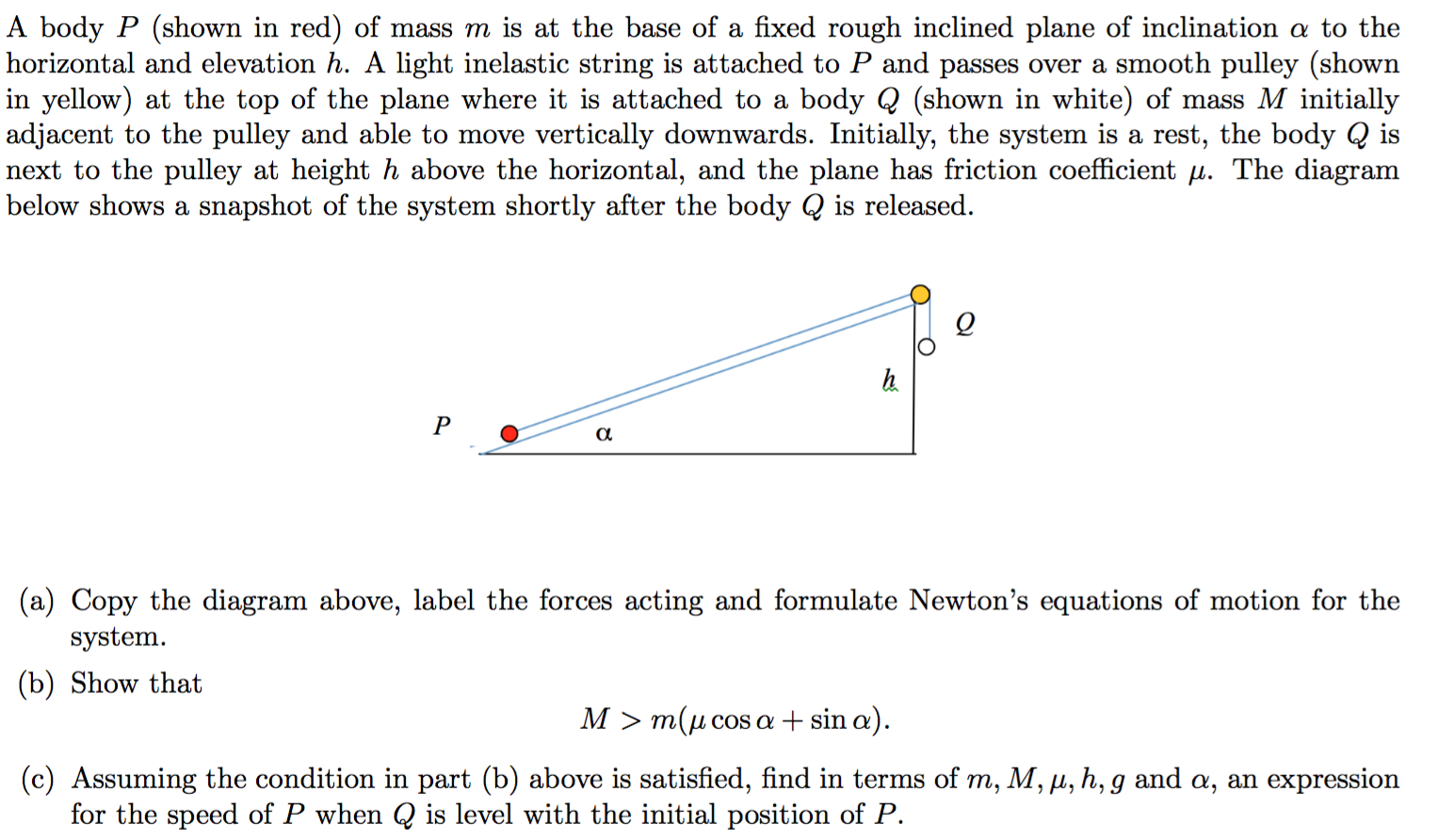A body P (shown in red) of mass m is at the base of a fixed rough inclined plane of inclination a to the horizontal and elevation h. A light inelastic string is attached to P and passes over a smooth pulley (shown in yellow) at the top of the plane where it is attached to a body Q (shown in white) of mass M initially adjacent to the pulley and able to move vertically downwards. Initially, the system is a rest, the body Q is next to the pulley at height h above the horizontal, and the plane has friction coefficient . The diagram below shows a snapshot of the system shortly after the body Q is released. Po a (a) Copy the diagram above, label the forces acting and formulate Newton's equations of motion for the system. (b) Show that M > m(u cos a + sin a). (c) Assuming the condition in part (b) above is satisfied, find in terms of m, M, u, h, g and a, an expression for the speed of P when Q is level with the initial position of P.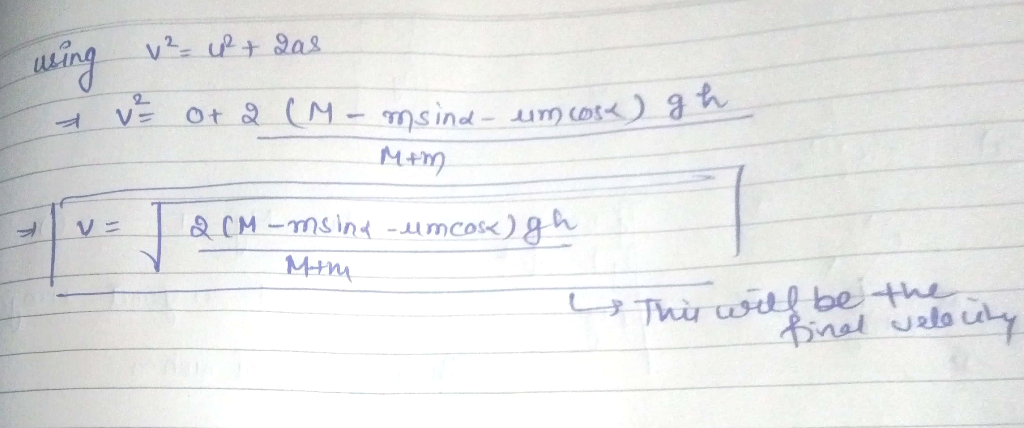#### Earn Coin

Coins can be redeemed for fabulous gifts.

Similar Homework Help Questions
• ### The axis of a smooth fixed ciular cylinder of radius R is horizontal. A particle of mass m is att...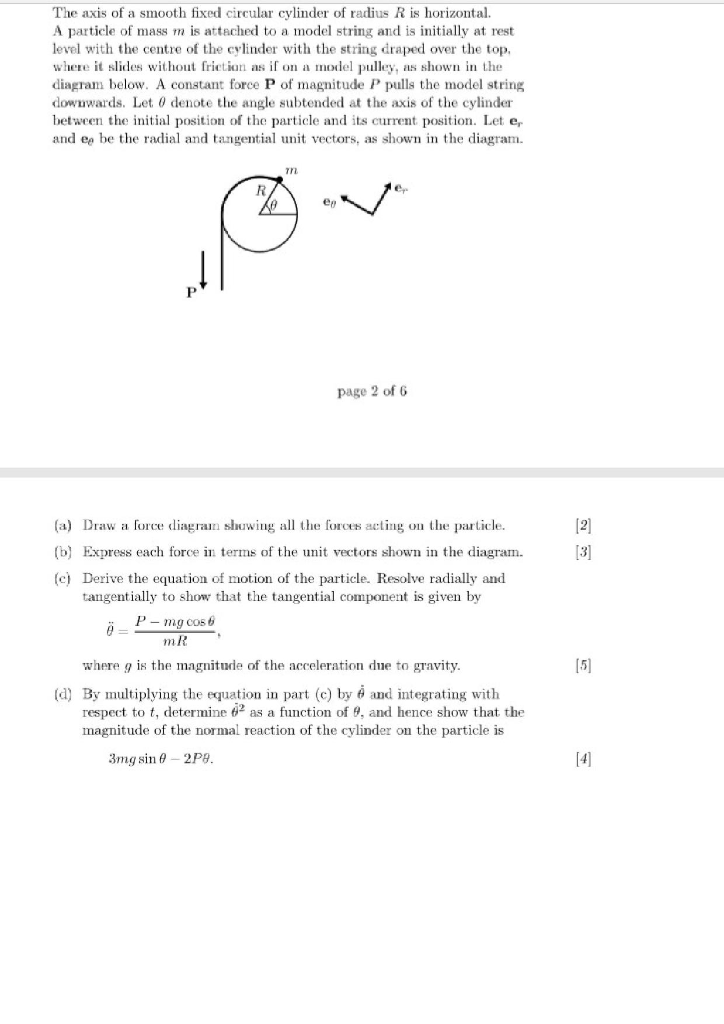The axis of a smooth fixed ciular cylinder of radius R is horizontal. A particle of mass m is attached to a model string and is initially at rest level with the cre of the cylinder with the string draped over the top, whee t slids without frictian as if on a model pulley, s shown in the diagram below. A constant force P of magnitude P pulls the model string downwards. Let 0 denote the angle subtended at the...

• ### 1&2 please. thanks 1. A 10 kg mass is hung from two light strings which pass...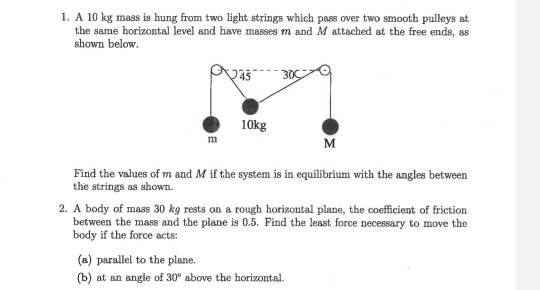1&2 please. thanks 1. A 10 kg mass is hung from two light strings which pass over two smooth pulleys at the same horizontal level and have masses m and M attached at the free ends, as shown below. 945 --3000 10kg Find the values of m and M if the system is in equilibrium with the angles between the strings as shown. 2. A body of mass 30 kg rests on a rough horizontal plane, the coefficient of friction...

• ### Please show all work with algebra. Problem 4 1. An object with a mass m is...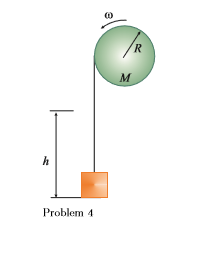Please show all work with algebra. Problem 4 1. An object with a mass m is attached to the free end of a light string wrapped around a reel of radius R and mass M. The reel is a solid disk, free to rotate in a vertical plane about the horizontal axis passing through its center (of mass) as shown in the figure below. The suspended object is released from rest h m above the floor. The entire system is...

• ### To apply the equations of motion to a system that involves rotation about a fixed axis and to use this information to determine key characteristics. The slender rod AB shown has a mass of m=57.0 kg an...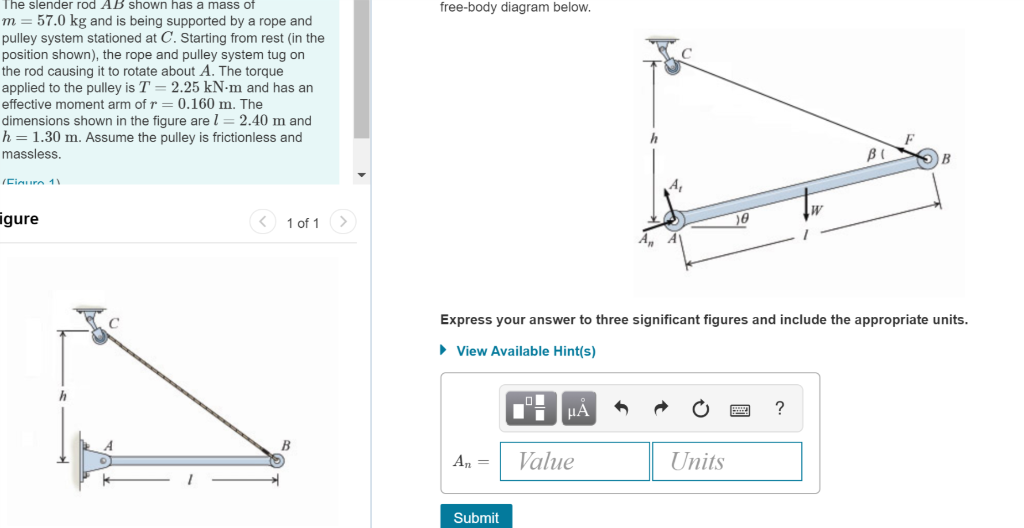To apply the equations of motion to a system that involves rotation about a fixed axis and to use this information to determine key characteristics. The slender rod AB shown has a mass of m=57.0 kg and is being supported by a rope and pulley system stationed at C. Starting from rest (in the position shown), the rope and pulley system tug on the rod causing it to rotate about A. The torque applied to the pulley is T=2.25 kN⋅m...

• ### In the system shown below, there is a block of mass M = 3.6 kg resting...

In the system shown below, there is a block of mass M = 3.6 kg resting on a horizontal ledge. The coefficient of kinetic friction between the ledge and the block is 0.25. The block is attached to a string that passes over a pulley, and the other end of the string is attached to a hanging block of mass m = 2.2 kg. The pulley is a uniform disk of radius 7.9 cm and mass 0.66 kg. Find the...

• ### 2. A uniform, solid cylinder with mass M and radius 2R is on an incline plane with angle of inclination of 6. A str...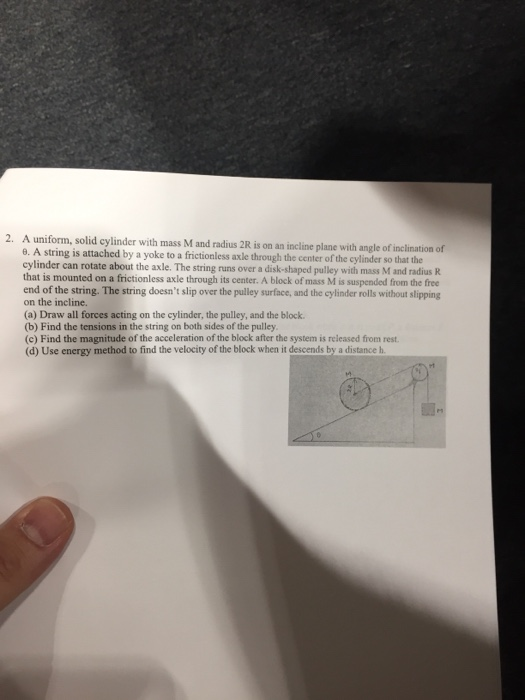2. A uniform, solid cylinder with mass M and radius 2R is on an incline plane with angle of inclination of 6. A string is attached by a yoke to a frictionless axle through the center of the cylinder so that the cylinder can rotate about the axle. The string runs over a disk-shaped pulley with mass M and radius R that is mounted on a frictionless axle through its center. A block of mass M is suspended from the...

• ### Problem 3.9 An inextensible, massless rope is supported by a pulley of negligible mass. Its end p...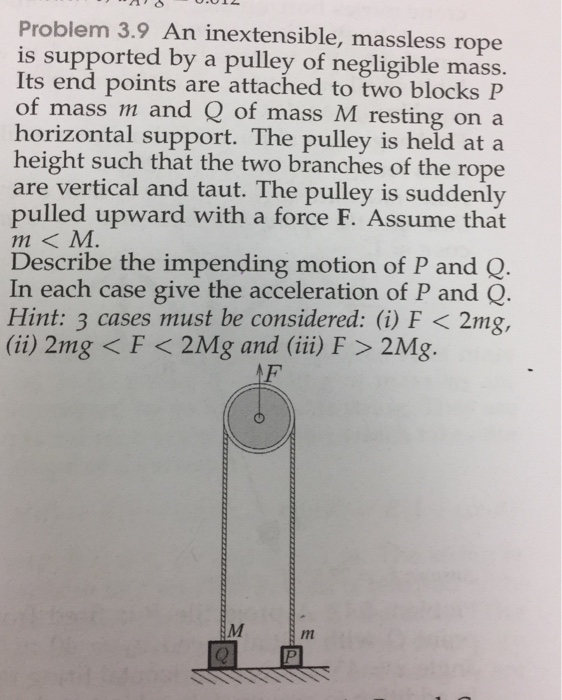Problem 3.9 An inextensible, massless rope is supported by a pulley of negligible mass. Its end points are attached to two blocks P of mass m and Q of mass M resting on a horizontal support. The pulley is held at height such that the two branches of the rope are vertical and taut. The pulley is suddenly pulled upward with a force F. Assume that m < M. Describe the impending motion of P and Q In each case...

• ### (a) As shown in Figure 4(a), a wooden block A with mass ma = 2.4 kg...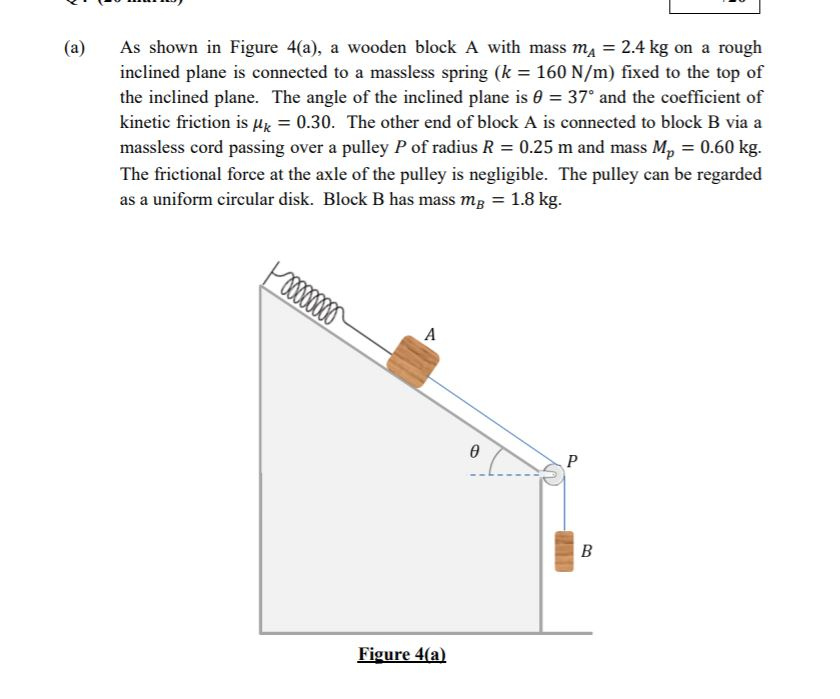(a) As shown in Figure 4(a), a wooden block A with mass ma = 2.4 kg on a rough inclined plane is connected to a massless spring (k = 160 N/m) fixed to the top of the inclined plane. The angle of the inclined plane is @ = 37º and the coefficient of kinetic friction is uk = 0.30. The other end of block A is connected to block B via a massless cord passing over a pulley P of...

• ### when it strikes the ground Free-Body Diagram: A pulley system slides down a hill. The hill is tilted up a' an a...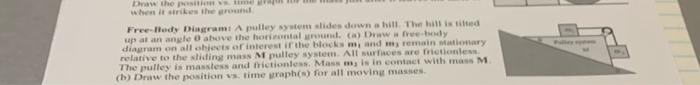when it strikes the ground Free-Body Diagram: A pulley system slides down a hill. The hill is tilted up a' an angle O above the horizontal ground, (a) Draw·free-kdy rest if the blocks m and m remain stationary relative to the sliding mass M pulley system. All surfaces are frictionless ulley is massless and frictionless. Mass m is in contact with mass M w the position vs. time graph(s) for all moving masses. when it strikes the ground Free-Body Diagram:...

• ### Frictionless plane M 1.) Consider the coupled system shown at the right. The mass M is...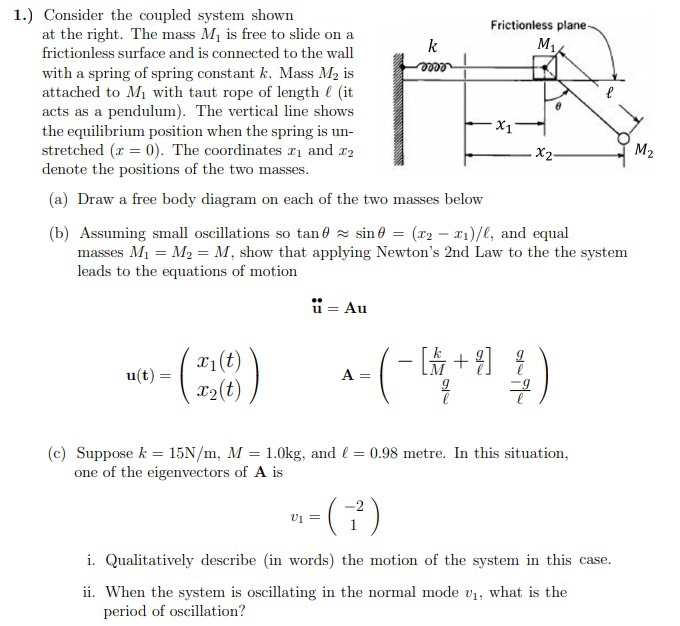Frictionless plane M 1.) Consider the coupled system shown at the right. The mass M is free to slide on a frictionless surface and is connected to the wall with a spring of spring constant k. Mass M2 is 2000 attached to My with taut rope of length (it acts as a pendulum). The vertical line shows the equilibrium position when the spring is un- stretched (r = 0). The coordinates 21 and 12 denote the positions of the two...

Free Homework Help App